# Chapter 2 : Basics of Electricity

### Topics covered in this snack-sized chapter:

#### Coulomb’s Law arrow_upward

###### Coulomb’s Law states that:

• The magnitude of the Electrostatic force of interaction between two point charges is:
• Directly proportional to the scalar multiplication of the magnitude of charges and,
• Inversely proportional to the square of the distance between them.
• The electric force F acting on a point charge Q1 as a result of the presence of a second point charge Q2 is given by:
•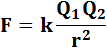• Here, k is Coulomb’s Constant
•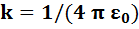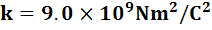#### Ohm’s Law arrow_upward

• Ohm’s Law states that, “The resistance of a conductor is equal to ratio of voltage over current”.
•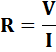• It can also be presented as:
• “When electrical potential (voltage) creates a flow of electricity (current), the current and the electrical resistance of the circuit are proportional to the voltage.”
•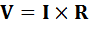• Here,
• R= Resistance
• I = Current
• V= Voltage

#### Gauss Law arrow_upward

• “The flux of electric field through a closed surface is proportional to the charge enclosed”.
•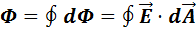• Electric Flux is the amount of electric field passing through a closed surface.
• Flux is positive when electric field is outward, and
• Negative when electric field is inward (through the closed surface).

#### Electrical Source arrow_upward

• An Electrical Source is a voltage or current generator capable of supplying energy to a circuit.
• Electrical sources, in practice, include things like:
• Batteries
• The ever-present wall plug
• Power supplies

#### Voltage Source arrow_upward

###### Ideal Voltage Source:

• An ideal voltage source is a circuit element where the voltage across the source is independent of the current through it.
• The internal resistance of an ideal voltage source is zero.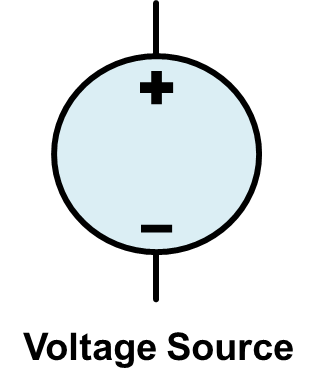###### Ideal Independent Voltage Source:

• An ideal independent voltage source is characterized by having a constant voltage across its terminals, regardless of the load connected to the terminals.
• The ideal voltage source can supply any amount of current.
• Furthermore, the ideal independent voltage source can supply any amount of power.

###### Dependent Voltage Source:

• A dependent voltage source is characterized by depending on a voltage or current somewhere else in the circuit.
• The symbol for the voltage source is shown below: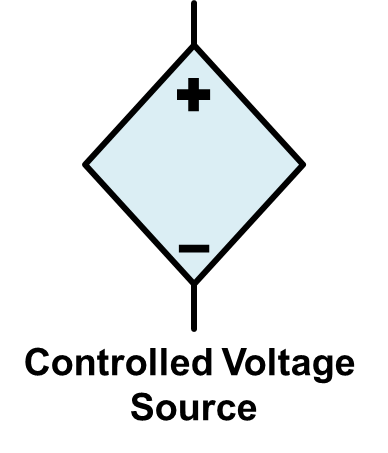• A circuit containing a dependent voltage source is shown below:
•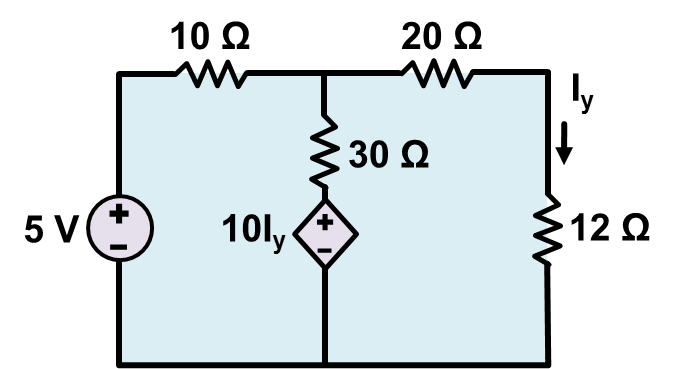#### Current Source arrow_upward

###### Ideal Current Source:

• An ideal current source is a circuit element where the current through the source is independent of the voltage across it.
• The internal resistance of an ideal current source is infinite.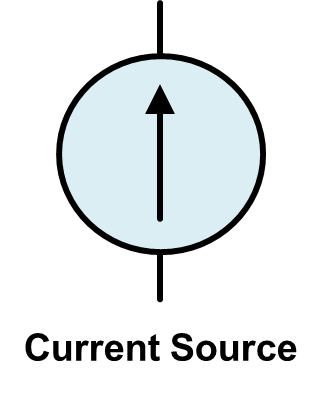###### Ideal Independent Current Source:

• An ideal independent current source is characterized by providing a constant value of current, regardless of the load.
• If the current source is truly ideal, it can provide any value of voltage and any amount of power.

###### Dependent Current Source:

• A dependent current source is characterized by depending on a voltage or current somewhere else in the circuit.
•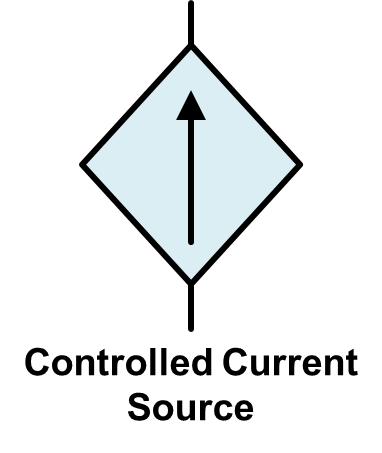• A circuit containing a dependent current source is shown below:
• A circuit with a voltage controlled dependent current source.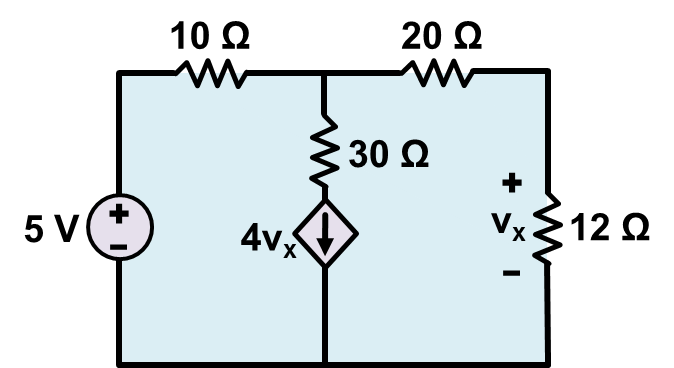#### Thank You from Kimavi arrow_upward

• Please email us at admin@kimavi.com and help us improve this tutorial.